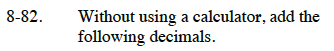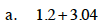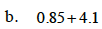Home > CC1 > Chapter 8 > Lesson 8.2.1 > Problem8-82

8-82.
1.Without a calculator, add the following decimals. Homework Help ✎

1. 1.2 + 3.04

2. 0.85 + 4.1Think about the place values of each of the digits and add like parts. In the first number, the 1 means 1 whole and the 3 means 3 wholes, so adding them, we get 4 wholes.

Now we can add the total number of hundreds. In the first number, there are 2 tenths, or 20 hundreds. In the second number, there are only 4 hundredths. So combined we have 20 + 4 = 24 hundreds.

4 wholes and 24 hundredths is written 4.24.Follow the same steps as in part (a).